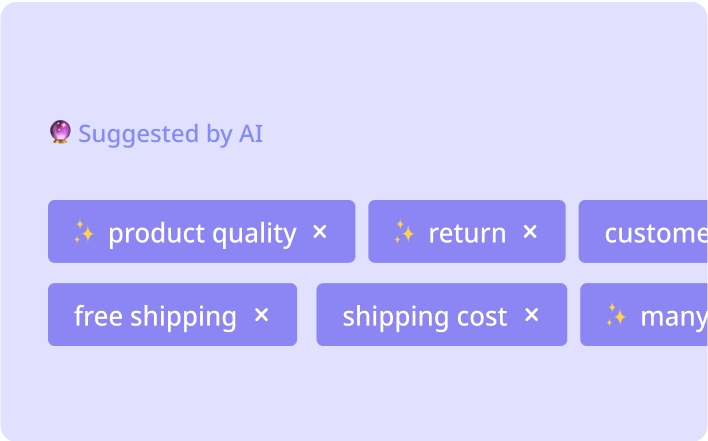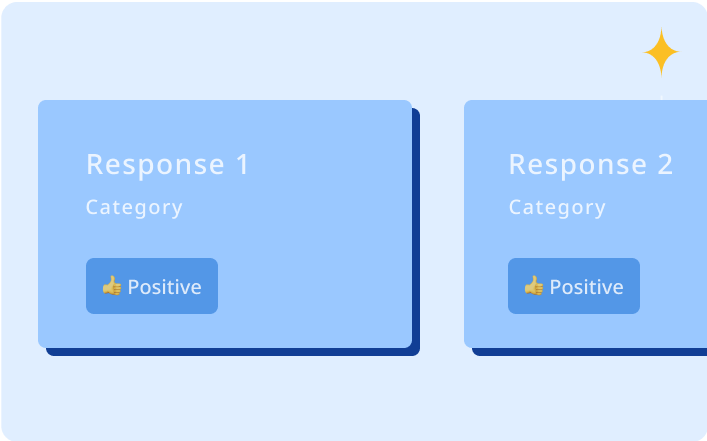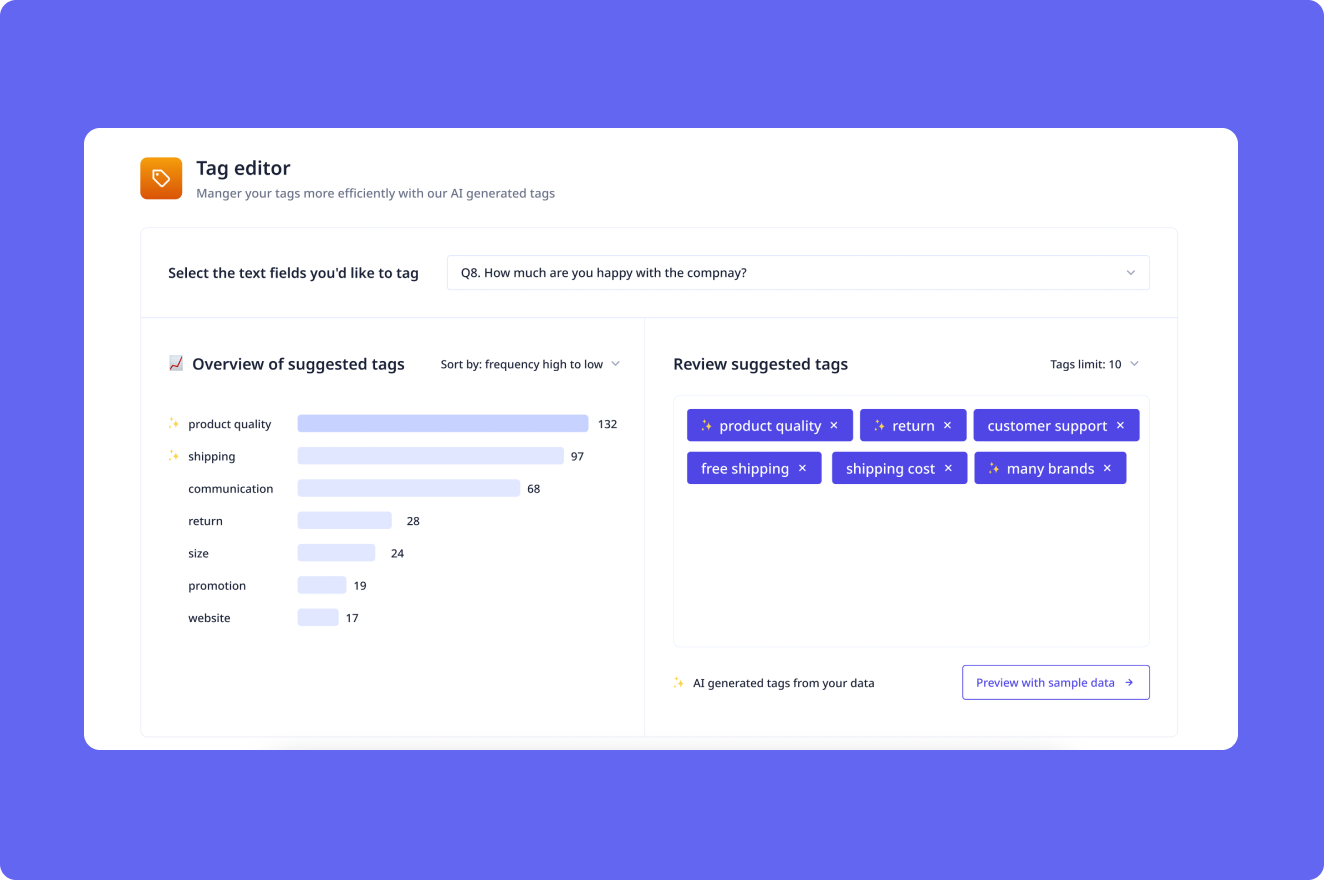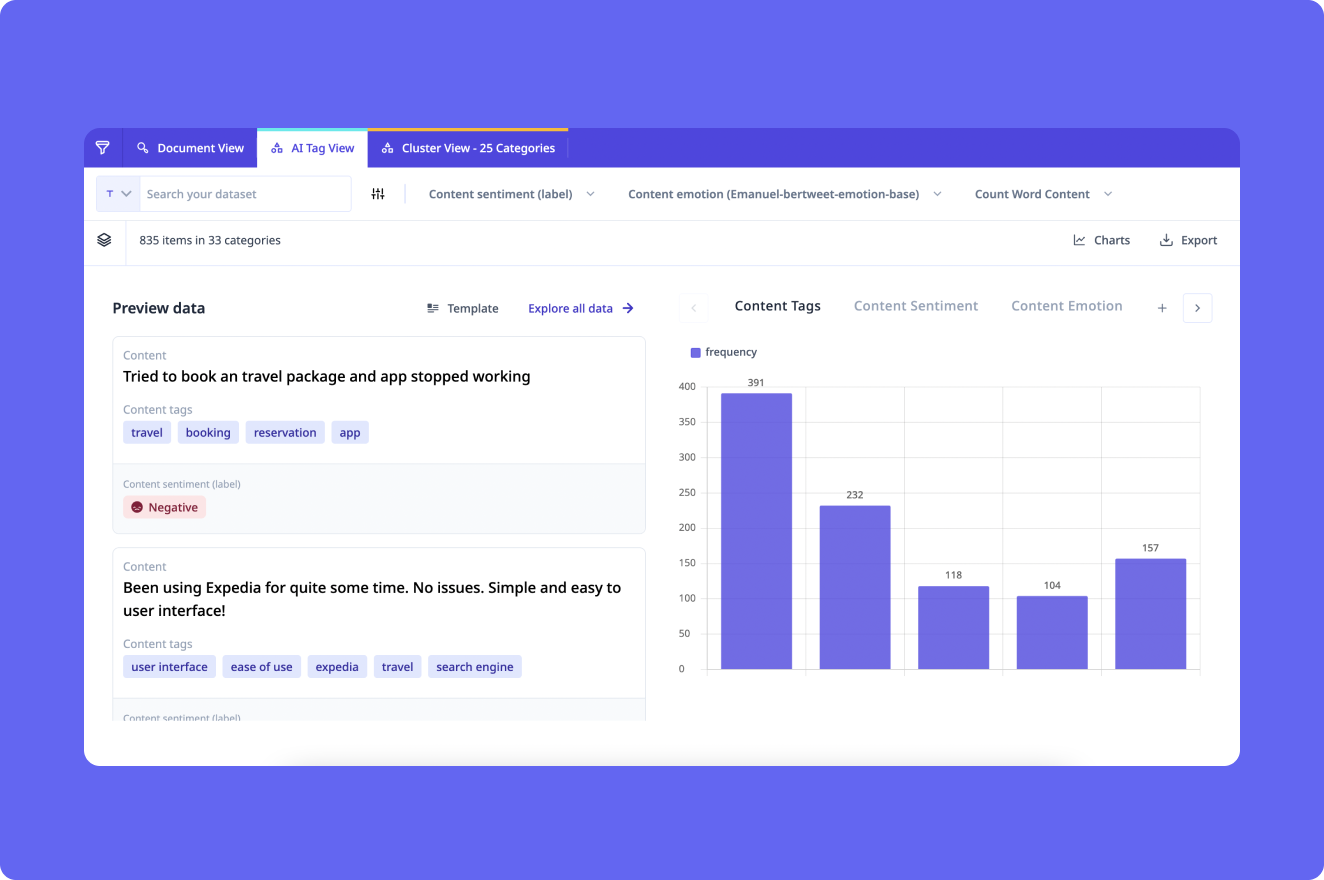# Unlock the Power of Excel Formulas!

Make Excel formulas easier to understand with Excel Formula Explain! Our workflow takes complex formulas and breaks them down into plain English, helping you get the most out of your data. Try it today!
Enabling AI for the world’s best, from startups to publicly listed.## Quickly explain Excel formulas.

Discover how Excel Formula Explain can quickly and easily explain complex Excel formulas in plain English!

## Designed for every team### Uncover the benefits of quickly understanding complex Excel formulas to save time and improve accuracy!### Unlock the power of Excel Formula Explain to make complex Excel formulas more understandable and maximize your spreadsheet productivity!### Bring your data to life. Start free.With Relevance AISave time and money by quickly understanding complex Excel formulas.Increase efficiency by instantly understanding Excel formulas and quickly analyzing data.Improve accuracy by having Excel formulas explained in plain languageWithout Relevance AISave time and money by quickly understanding complex Excel formulas.Low efficiency due to difficulty understanding Excel formulas and analyzing data.High risk of errors due to lack of understanding of Excel formulas.

## Example use cases### Unlock the Power of Excel Formulas!